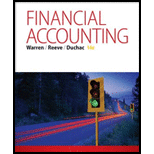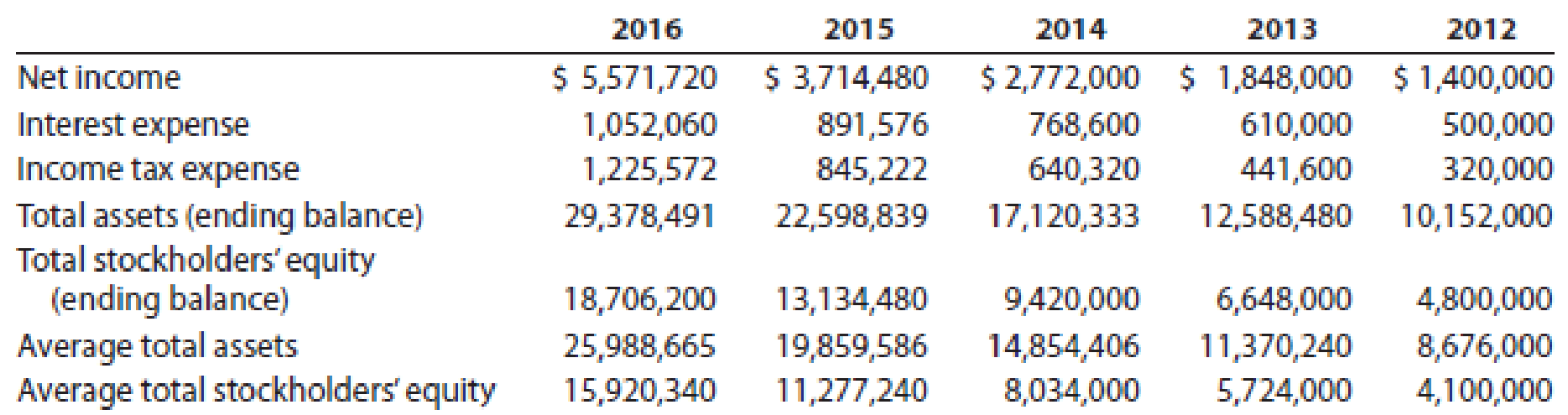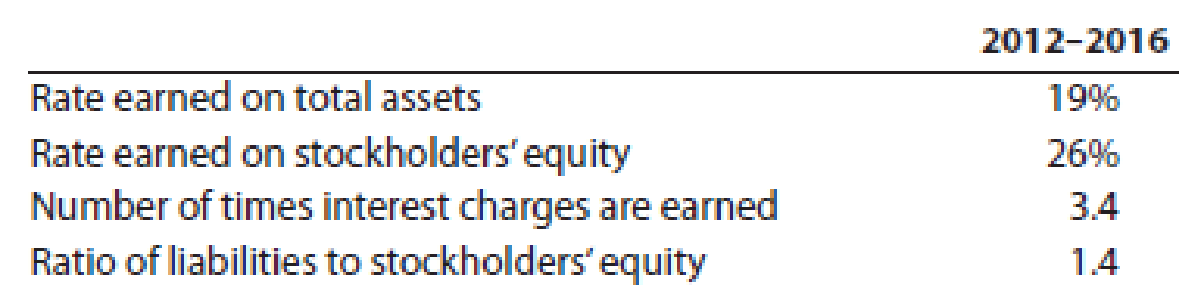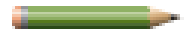# Crosby Company has provided the following comparative information: You have been asked to evaluate the historical performance of the company over the last five years. Selected industry ratios have remained relatively steady at the following levels for the last five years: Instructions 1. Prepare four line graphs with the ratio on the vertical axis and the years on the horizontal axis for the following four ratios (rounded to one decimal place): a. Rate earned on total assets b. Rate earned on stockholders’ equity c. Number of times interest charges are earned d. Ratio of liabilities to stockholders’ equity Display both the company ratio and the industry benchmark on each graph. That is, each graph should have two lines. 2. Prepare an analysis of the graphs in (1).### Financial Accounting

14th Edition
Carl Warren + 2 others
Publisher: Cengage Learning
ISBN: 9781305088436

#### Solutions

Chapter
Section### Financial Accounting

14th Edition
Carl Warren + 2 others
Publisher: Cengage Learning
ISBN: 9781305088436
Chapter 17, Problem 5PB
Textbook Problem
126 views

## Crosby Company has provided the following comparative information:You have been asked to evaluate the historical performance of the company over the last five years.Selected industry ratios have remained relatively steady at the following levels for the last five years:Instructions 1. Prepare four line graphs with the ratio on the vertical axis and the years on the horizontal axis for the following four ratios (rounded to one decimal place): a. Rate earned on total assets b. Rate earned on stockholders’ equity c. Number of times interest charges are earned d. Ratio of liabilities to stockholders’ equity Display both the company ratio and the industry benchmark on each graph. That is, each graph should have two lines. 2.Prepare an analysis of the graphs in (1).

1.  a.

To determine

Determine the Rate earned on total assets for five years and Display the determined ratio in a graph.

### Explanation of Solution

Financial Ratios: Financial ratios are the metrics used to evaluate the liquidity, capabilities, profitability, and overall performance of a company.

Return on assets determines the particular company’s overall earning power. It is determined by dividing sum of net income and interest expense and average total assets.

Formula:

Rate of return on assets=Netincome + Interest expenseAverage total assets

Rate earned on total assets for five years (2012 to 2016) is calculated as below:

Rate earned on total assets(2016)=Netincome + Interest expenseAverage total assets=$6,623,780$25,988,665=25.5%

Rate earned on total assets(2015)=Netincome + Interest expenseAverage total assets=$4,606,056$19,859,586=23

b)

To determine

Determine the Rate earned on stockholders’ equity for five years and Display the determined ratio in a graph.

c)

To determine

Determine number of times interest charges are earned ratio for five years and Display the determined ratio in a graph.

d)

To determine

Determine Ratio of liabilities to stockholders’ equity for five years and Display the determined ratio in a graph.

2.

To determine

Prepare analysis of graphs.

### Still sussing out bartleby?

Check out a sample textbook solution.

See a sample solution

#### The Solution to Your Study Problems

Bartleby provides explanations to thousands of textbook problems written by our experts, many with advanced degrees!

Get Started